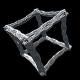# Pendulum Plots

This is a quick post showing an alternative way to display the result of plotting double, triple and quadruple pendulums.

Based on my previous magnetic pendulum simulation and plots I had the idea to try a similar plot style for the double, triple and quadruple pendulums.

If you simulate a pendulum attached to another pendulum in zero gravity without any friction you get a system that runs continuously and creates a plot like the following gif animation.With 3 pendulums the plot is more complex.With 4 it is even more complex.Another way to visualize this is to plot a 2D image with the X axis being 0 to 360 degrees of the first pendulum and the Y axis being 0 to 360 degrees of the second pendulum. The simulations are run for 5000 steps at each pixel location. Depending on which quadrant of the screen the pendulum is in after the 5000 steps determines the color of the pixel (red, green, blue or yellow). The top left pixel sets the pendulum angles to both 0, runs the simulation for 5000 steps and because the end of the two pendulums is located in the lower left quarter of the screen the pixel is colored green. This process is then repeated for the remaining pixels of the image.

Here is the result for the double pendulumand the tripleThe original code was taking 12 minutes for a 320×240 image using the simplest double pendulum code. I am patient, but not that patient. A quick recode into GLSL and the GPU took over the number crunching and now it is rendering 640×480 results in under 2 seconds.

Here is a gif animation of the double pendulum plot with the number of simulation iterations increasing each frame.The above plotting and shader code is now included with Visions Of Chaos if you just want to experiment without having to do any coding.

Jason.

## 2 responses to “Pendulum Plots”

1.Narim says:

Hi Jason can i use this gif for a video?

•softologyblog says:

Yes you can. Send me a link to the video when done.